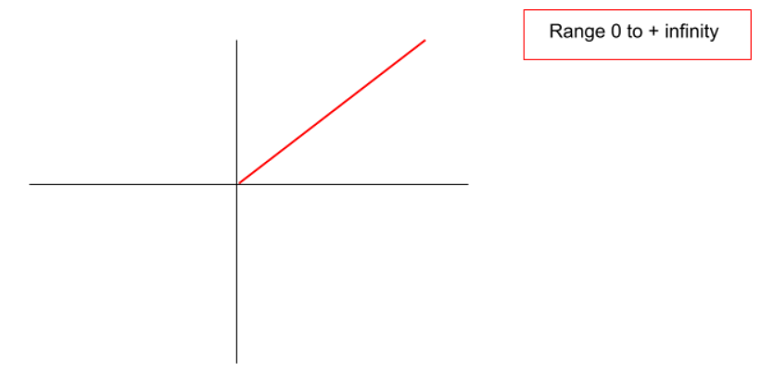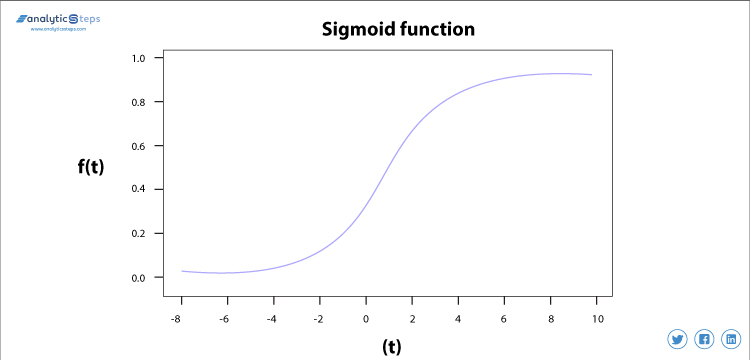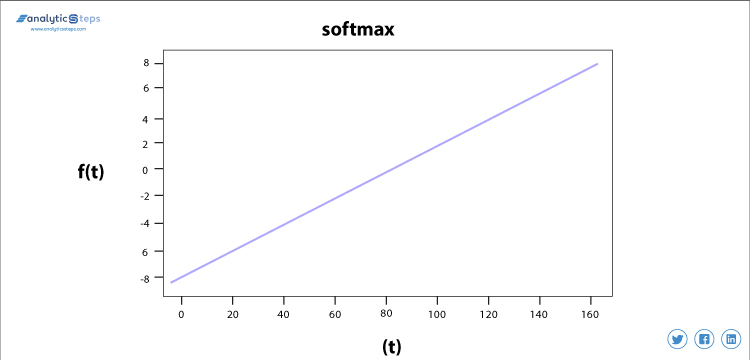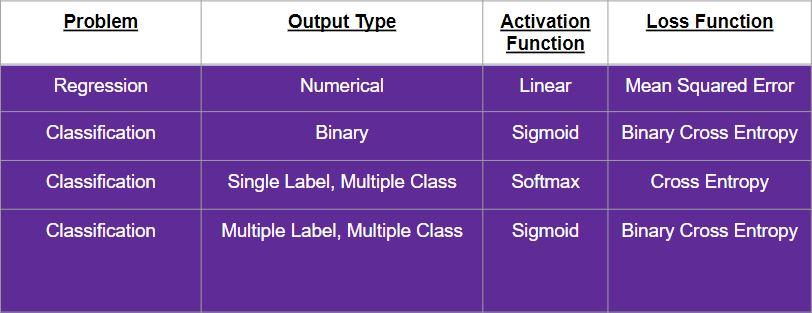• Category
• >Deep Learning
• >Machine Learning

# How to decide which Activation Function and Loss Function to use?

• Rohit Dwivedi
• Jun 13, 2020
• Updated on: Jul 02, 2021## Introduction

The motive of the blog is to give you some ideas on the usage of “Activation Function” & “Loss function” in different scenarios. I assume you have a fair idea about activation functions and loss functions.

Choosing an activation function and loss function is directly dependent upon the output you want to predict. There are different cases and different outputs of a predictive model. Before I introduce you to such cases let see an introduction to the activation function and loss function.

### Activation Function

The activation function activates the neuron that is required for the desired output, converts linear input to non-linear output.

In neural networks, activation functions, also known as transfer functions, define how the weighted sum of the input can be transformed into output via nodes in a layer of networks. They are treated as a crucial part of neural networks’ design.

In hidden layers, the selection of activation function controls how perfectly a network model works to learn the training dataset while in the output layer, it determines the types of predictions a model can generate.

If you are not aware of the different activation functions I would recommend you visit here to get an in-depth explanation of different types of activation functions here.

### Loss Function

Loss function helps you figure out the performance of your model in prediction, how good the model is able to generalize. It computes the error for every training.

The loss function is the function calculating the distance between the current output and the expected output of the algorithm. The function can also evaluate how an algorithm models the data and can be categorized into two categories

• Classification for discrete values

• Regression for continuous values.

You can read more about loss functions and how to reduce the loss here.

It is said that the goal decides how to validate the performance of the business. Similarly, output decides what loss function and activation function is to be used.

## Different Cases to Adopt Activation Function and Loss Function

### CASE 1:

#### When the output is a numerical value that you are trying to predict

Consider predicting the prices of houses provided with different features of the house. A neural network structure where the final layer or the output later will consist of only one neuron that reverts the numerical value. For computing the accuracy score the predicted values are compared to true numeric values.

Activation Function to be used in such cases,

• Linear Activation - This type of activation function gives the output in a numeric form that is the demand for this case.Linear Activation Function, Source

• ReLU Activation - This activation function gives you positive numeric outputs as a result.ReLu Activation Function

Loss function to be used in such cases,

• Mean Squared Error (MSE) - This loss function is responsible to compute the average squared difference between the true values and the predicted values.

(Must read: Cost Function in machine learning)

### CASE 2:

#### When the output you are trying to predict is Binary

Consider a case where the aim is to predict whether a loan applicant will default or not. In these types of cases, the output layer consists of only one neuron that is responsible to result in a value that is between 0 and 1 that can be also called probabilistic scores.

For computing the accuracy of the prediction, it is again compared with the true labels. The true value is 1 if the data belongs to that class or else it is 0.

(Suggested blog: Perceptron Model in Machine Learning)

Activation Function to be used in such cases,

• Sigmoid Activation -  This activation function gives the output as 0 and 1.Sigmoid Activation Function

Loss function to be used in such cases,

• Binary Cross Entropy - The difference between the two probability distributions is given by binary cross-entropy. (p,1-p) is the model distribution predicted by the model, to compare it with true distribution, the binary cross-entropy is used.

(Suggested blog: Cross-validation in machine learning)

### CASE 3:

#### Predicting a single class from many classes

Consider a case where you are predicting the name of the fruit amongst 5 different fruits. In this case, the output layer will consist of only one neuron for every class and it will revert a value between 0 and 1, the output is the probability distribution that results in 1 when all are added.

Each output is checked with its respective true value to get the accuracy. These values are one-hot-encoded which means if will be 1 for the correct class or else for others it would be zero.

Activation Function to be used in such cases,

• Softmax Activation -  This activation function gives the output between 0 and 1 that are the probability scores which if added gives the result as 1.Softmax Activation Function

Loss function to be used in such cases,

• Cross-Entropy - It computes the difference between two probability distributions.

• (p1,p2,p3) is the model distribution that is predicted by the model where p1+p2+p3=1. This is compared with the true distribution using cross-entropy.

(Read also: Decision tree algorithms in machine learning)

### CASE 4:

#### Predicting multiple labels from multiple class

Consider the case of predicting different objects in an image having multiple objects. This is termed a multiclass classification. In these types of cases, the output layer consists of only one neuron that is responsible to result in a value that is between 0 and 1 that can be also called probabilistic scores.

For computing the accuracy of the prediction, it is again compared with the true labels. The true value is 1 if the data belongs to that class or else it is 0.

Activation Function to be used in such cases,

• Sigmoid Activation -  This activation function gives the output as 0 and 1.Sigmoid Activation Function

Loss function to be used in such cases,

• Binary Cross Entropy - The difference between the two probability distributions is given by binary cross-entropy. (p,1-p) is the model distribution predicted by the model, to compare it with true distribution, the binary cross-entropy is used.

## Outline

The below table concludes to quickly check which activation and loss function to use in different problem statements and desired outputs.Activation and Loss Function

## Conclusion

It is very important to check which activation function and loss function is to be used in different problem scenarios in machine learning models or deep learning modelsOften people get confused about the usage of these functions. I have tried to give you an idea when to which type of activation and loss functions.

I have discussed different cases where the output is either binary, numerical, single label or multiple labels and corresponding activation and loss functions to be used. You can also check documentation where different activation functions are explained with the code here and there are other loss functions also that are used to compute loss; you can refer to them here